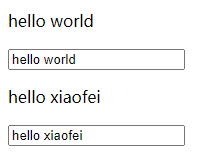﻿ vue 数据双向绑定的实现方法_vue.js_脚本之家
vue.js# vue 数据双向绑定的实现方法

## 1. 前言## 2. 代码实现

### 2.1 目的分析```<div id="app">
<h1 v-text="msg"></h1>
<input type="text" v-model="msg">
<div>
<h1 v-text="msg2"></h1>
<input type="text" v-model="msg2">
</div>
</div>```
```let vm = new Vue({
el: "#app",
data: {
msg: "hello world",
msg2: "hello xiaofei"
}
})```

• 第一步：将data中的数据同步到页面上，实现 M ==> V 的初始化；
• 第二步：当input框中输入值时，将新值同步到data中，实现 V ==> M 的绑定；
• 第三步：当data数据发生更新的时候，触发页面发生变化，实现 M ==> V 的绑定。

### 2.2 实现过程

#### 2.2.1 入口代码

```class Vue {
// 接收传进来的对象
constructor(options) {
// 保存有效信息
this.\$el = document.querySelector(options.el);
this.\$data = options.data;

// 容器: {属性1: [wathcer1, wathcer2...], 属性2: [...]}，用来存放每个属性观察者
this.\$watcher = {};

// 解析元素: 实现Compile
this.compile(this.\$el); // 要解析元素, 就得把元素传进去

// 劫持数据: 实现 Observer
this.observe(this.\$data); // 要劫持数据, 就得把数据传入
}
compile() {}
observe() {}
}```

#### 2.2.2 页面初始化

• 首先要获取到el下面的所有子节点，然后遍历这些子节点，如果子节点还有子节点，那我们就需要用到递归的思想；
• 遍历子节点找到所有有指令的元素，并将对应的数据渲染到页面中。

```class Vue {
// 接收传进来的对象
constructor(options) {
// 获取有用信息
this.\$el = document.querySelector(options.el);
this.\$data = options.data;

// 容器: {属性1: [wathcer1, wathcer2...], 属性2: [...]}
this.\$watcher = {};

// 2. 解析元素: 实现Compile
this.compile(this.\$el); // 要解析元素, 就得把元素传进去

// 3. 劫持数据: 实现 Observer
this.observe(this.\$data); // 要劫持数据, 就得把数据传入
}
compile(el) {
// 解析元素下的每一个子节点, 所以要获取el.children
// 备注: children 返回元素集合, childNodes返回节点集合
let nodes = el.children;

// 解析每个子节点的指令
for (var i = 0, length = nodes.length; i < length; i++) {
let node = nodes[i];
// 如果当前节点还有子元素, 递归解析该节点
if(node.children){
this.compile(node);
}
// 解析带有v-text指令的元素
if (node.hasAttribute("v-text")) {
let attrVal = node.getAttribute("v-text");
node.textContent = this.\$data[attrVal]; // 渲染页面
}
// 解析带有v-model指令的元素
if (node.hasAttribute("v-model")) {
let attrVal = node.getAttribute("v-model");
node.value = this.\$data[attrVal];
}
}
}
observe(data) {}
}
```#### 2.2.3 视图影响数据

```class Vue {
constructor(options) {
this.\$el = document.querySelector(options.el);
this.\$data = options.data;

this.\$watcher = {};

this.compile(this.\$el);

this.observe(this.\$data);
}
compile(el) {
let nodes = el.children;

for (var i = 0, length = nodes.length; i < length; i++) {
let node = nodes[i];
if(node.children){
this.compile(node);
}
if (node.hasAttribute("v-text")) {
let attrVal = node.getAttribute("v-text");
node.textContent = this.\$data[attrVal];
}
if (node.hasAttribute("v-model")) {
let attrVal = node.getAttribute("v-model");
node.value = this.\$data[attrVal];
// 看这里！！只多了三行代码！！
this.\$data[attrVal] = ev.target.value;
// 可以试着在这里执行:console.log(this.\$data),
// 就可以看到每次在输入框输入文字的时候,data中的msg值也发生了变化
})
}
}
}
observe(data) {}
}```

#### 2.2.4 数据影响视图

1) 我们将要实现一个 Wathcer 类，它有一个update方法，用来更新页面。观察者的代码如下：

```class Watcher{
constructor(node, updatedAttr, vm, expression){
// 将传进来的值保存起来，这些数据都是渲染页面时要用到的数据
this.node = node;
this.updatedAttr = updatedAttr;
this.vm = vm;
this.expression = expression;
this.update();
}
update(){
this.node[this.updatedAttr] = this.vm.\$data[this.expression];
}
}```

2) 试想，我们该给哪些数据添加观察者？何时给数据添加观察者？3) 劫持数据：利用对象的访问器属性getter和setter做到当数据更新的时候，触发一个动作，这个动作的主要目的就是让所有观察了该数据的观察者执行update方法。

1. 实现一个Wathcer类；
2. 在解析指令的时候（即在compile方法中）添加观察者；
3. 实现数据劫持（实现observe方法）。

```  class Vue {
// 接收传进来的对象
constructor(options) {
// 获取有用信息
this.\$el = document.querySelector(options.el);
this.\$data = options.data;

// 容器: {属性1: [wathcer1, wathcer2...], 属性2: [...]}
this.\$watcher = {};

// 解析元素: 实现Compile
this.compile(this.\$el); // 要解析元素, 就得把元素传进去

// 劫持数据: 实现 Observer
this.observe(this.\$data); // 要劫持数据, 就得把数据传入
}
compile(el) {
// 解析元素下的每一个子节点, 所以要获取el.children
// 拓展: children 返回元素集合, childNodes返回节点集合
let nodes = el.children;

// 解析每个子节点的指令
for (var i = 0, length = nodes.length; i < length; i++) {
let node = nodes[i];
// 如果当前节点还有子元素, 递归解析该节点
if (node.children) {
this.compile(node);
}
if (node.hasAttribute("v-text")) {
let attrVal = node.getAttribute("v-text");
// node.textContent = this.\$data[attrVal];
// Watcher在实例化时调用update, 替代了这行代码

/**
* 试想Wathcer要更新节点数据的时候要用到哪些数据?
* e.g.   p.innerHTML = vm.\$data[msg]
* 所以要传入的参数依次是: 当前节点node, 需要更新的节点属性, vue实例, 绑定的数据属性
*/
// 往容器中添加观察者: {msg1: [Watcher, Watcher...], msg2: [...]}
if (!this.\$watcher[attrVal]) {
this.\$watcher[attrVal] = [];
}
this.\$watcher[attrVal].push(new Watcher(node, "innerHTML", this, attrVal))
}
if (node.hasAttribute("v-model")) {
let attrVal = node.getAttribute("v-model");
node.value = this.\$data[attrVal];

this.\$data[attrVal] = ev.target.value;
})

if (!this.\$watcher[attrVal]) {
this.\$watcher[attrVal] = [];
}
// 不同于上处用的innerHTML, 这里input用的是vaule属性
this.\$watcher[attrVal].push(new Watcher(node, "value", this, attrVal))
}
}
}
observe(data) {
Object.keys(data).forEach((key) => {
let val = data[key];  // 这个val将一直保存在内存中,每次访问data[key],都是在访问这个val
Object.defineProperty(data, key, {
get() {
return val;  // 这里不能直接返回data[key],不然会陷入无限死循环
},
set(newVal) {
if (val !== newVal) {
val = newVal;// 同理,这里不能直接对data[key]进行设置,会陷入死循环
this.\$watcher[key].forEach((w) => {
w.update();
})
}
}
})
})
}
}

class Watcher {
constructor(node, updatedAttr, vm, expression) {
// 将传进来的值保存起来
this.node = node;
this.updatedAttr = updatedAttr;
this.vm = vm;
this.expression = expression;
this.update();
}
update() {
this.node[this.updatedAttr] = this.vm.\$data[this.expression];
}
}

let vm = new Vue({
el: "#app",
data: {
msg: "hello world",
msg2: "hello xiaofei"
}
})```